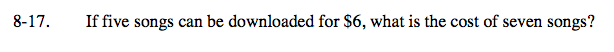Home > CC2MN > Chapter cc28 > Lesson cc28.1.1 > Problem8-17

8-17.

If five songs can be downloaded for $6, what is the cost of seven songs? Homework Help ✎How is the cost related to the number of songs? Set up a proportion relating the cost of the songs to the number of songs. $\frac{5}{6}=\frac{7}{\textit{x}}$ Cross multiply and divide to find the unknown value.$8.40